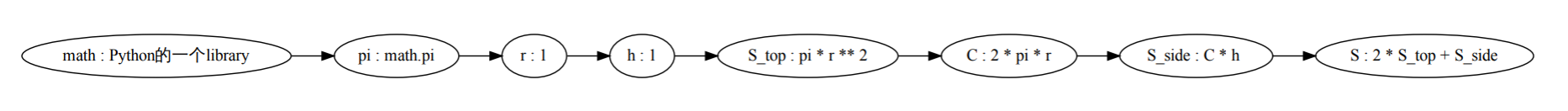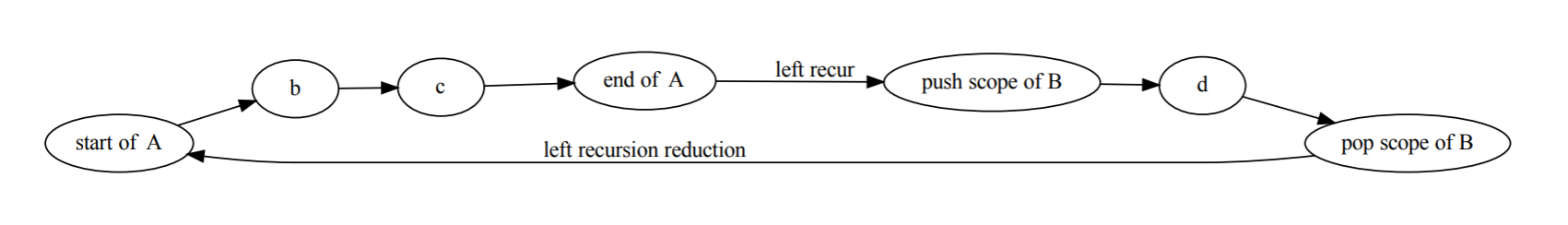# 我和Python的故事¶

## 第0节¶

Moshmosh 的正式Release, 对我来说有一些意义.

## 第2节: 转型的经过, 走向程序语言设计领域¶

４月, 在一个叫泰迪杯的比赛期间, 某种神秘的感觉降临了我.

## 第3节: 想要给Python添加高级语言特性的理想¶

# 圆柱面积 / surface area of a cylinder
from math import pi
r = 1  # the radius
h = 10 # the height

S = (2*S_top + S_side) where:
S_top  = pi*r**2
S_side = C * h where:
C = 2*pi*r


从 math 库中导入 pi

底面积 = (pi * 半径)的平方
测面积 = 周长 * 高, 其中
周长 = 2 * pi * 半径


# 表面积 = 2 * 底面积 + 侧面积
S = 2*S_top + S_side


从 math 库中导入 pi

底面积 = (pi * 半径)的平方
测面积 = 周长 * 高, 其中
周长 = 2 * pi * 半径A -> b c
A -> B d
B -> A eD, E, F, G, L, M, N, O 已知
A = f_bc(B, C) where
B = f_de(D, E)
C = f_gh(F, G)
H = f_ij(I, J) where
I = f_lm(L, M)
J = f_no(N, O)


D, E, F, G, L, M, N, O 已知
B = f_de(D, E)
C = f_gh(F, G)
A = f_bc(B, C)
I = f_lm(L, M)
J = f_no(N, O)
H = f_ij(I, J)


## 第4节: Flowpython¶

CPython的codebase很大, 我并不能一下找到我想要的全部文件(比如ast.c, symtable.c, Python-asdl, Grammar).

Flowpython最终是不实用的, 除开技术深度和设计的问题, 原因还有, 它过于”重”, 也不是portable的.

## 第4节: BNF, Parser Generator和语言制造机器¶

BNF和后续的很多故事都有关.

CPython项目中的 Grammar , 我是秒懂的, 没有任何学习的过程. 我只是浏览了一遍文件, 就了解了EBNF的各种语言构造.

[Int, *String, Float]
[1, "2", "2", 3.0]


• 一组基本的pattern: match(Int, 1) == True, match(Float, 3.0)

• pattern之间的组合方式: match([A, B, C], [a, b, c]) == True, 当 match(A, a) == match(B, b) == match(C, c) == True